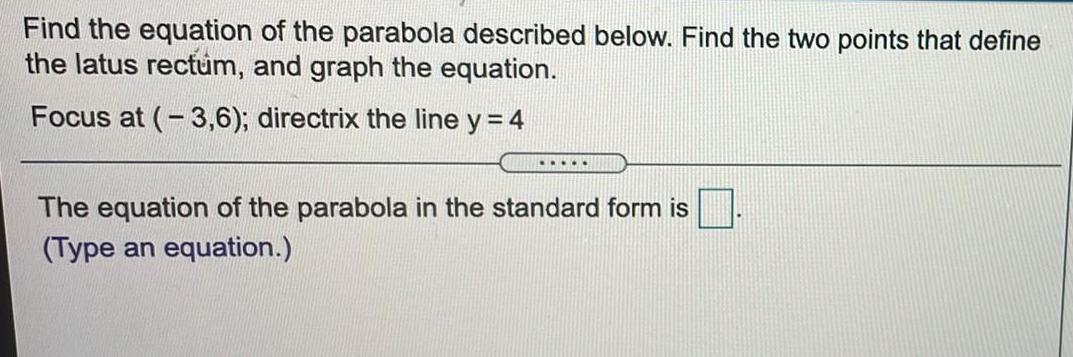Question:

# Find the equation of the parabola described below. Find the

Last updated: 8/8/2022Find the equation of the parabola described below. Find the two points that define the latus rectum, and graph the equation. Focus at (-3,6); directrix the line y = 4 The equation of the parabola in the standard form is (Type an equation.)